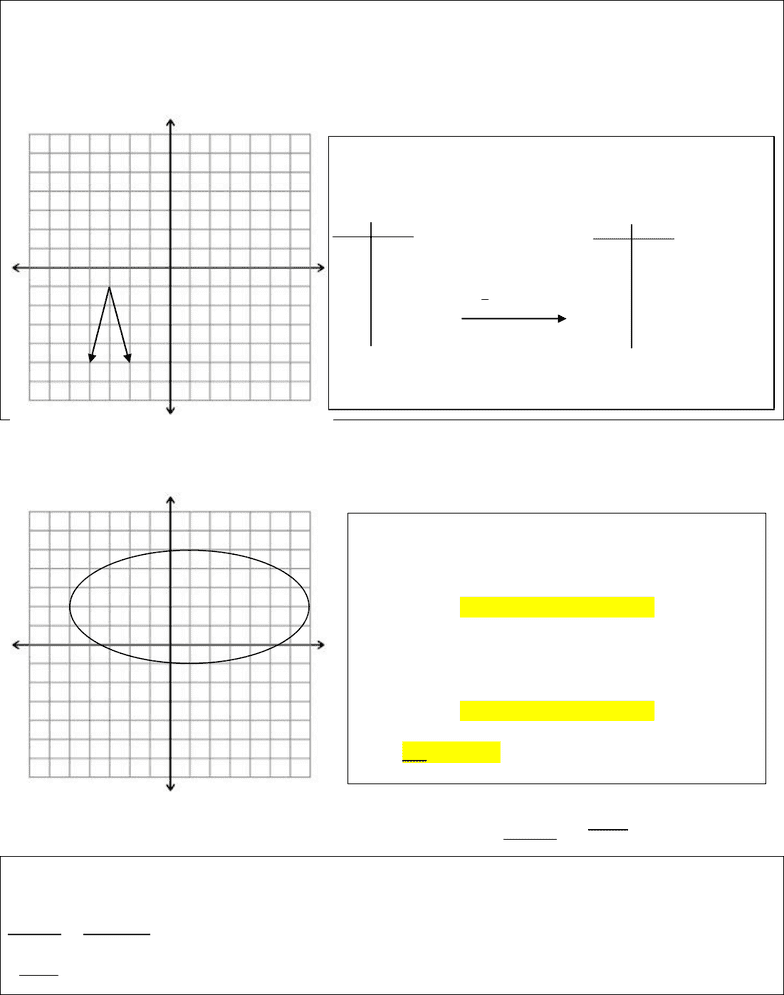Study Guides (380,000)
CA (150,000)
Carleton (5,000)
MATH (200)
Quiz

# MATH 1007 Quiz: Tutorial 1 - Solutions

Department
Mathematics
Course Code
MATH 1007
Professor
Kyle Harvey
Study Guide
Quiz

This preview shows page 1. to view the full 4 pages of the document.Question 1: Graph the following function:     
We write out the transformation formula:     , which means we should factor this to place it in the
correct form:     
Here we see that:
a =-2, b=2, c=-3, d=-1
Question 2: Determine the domain & range of the following graph. Also state whether the graph represents a
function. (Assume that the each grid is 1 unit).
Question 3: Determine the domain of the following equation:
   
Looking at each piece one at a time, we get:

 which means we cannot divide by 0, this means  .
   we cannot square root a negative, this means that    or   
Scanning left to right, we see that the only inputs that are
picked up are from -5 until 7. This gives us the domain:
        
Scanning bottom to top, we see that the only outputs
that are picked up are from -1 until 5. This gives us the
range:
         
This is not a function as it fails the vertical line test.
We can make our table of values and apply the transformations:
Parent function:   
x y x y
-2 2 -4 -5
-1 1
  -3.5 -3
0 0    -3 -1
1 1 -2.5 -3
2 2 -2 -5
###### You're Reading a Preview

Unlock to view full version